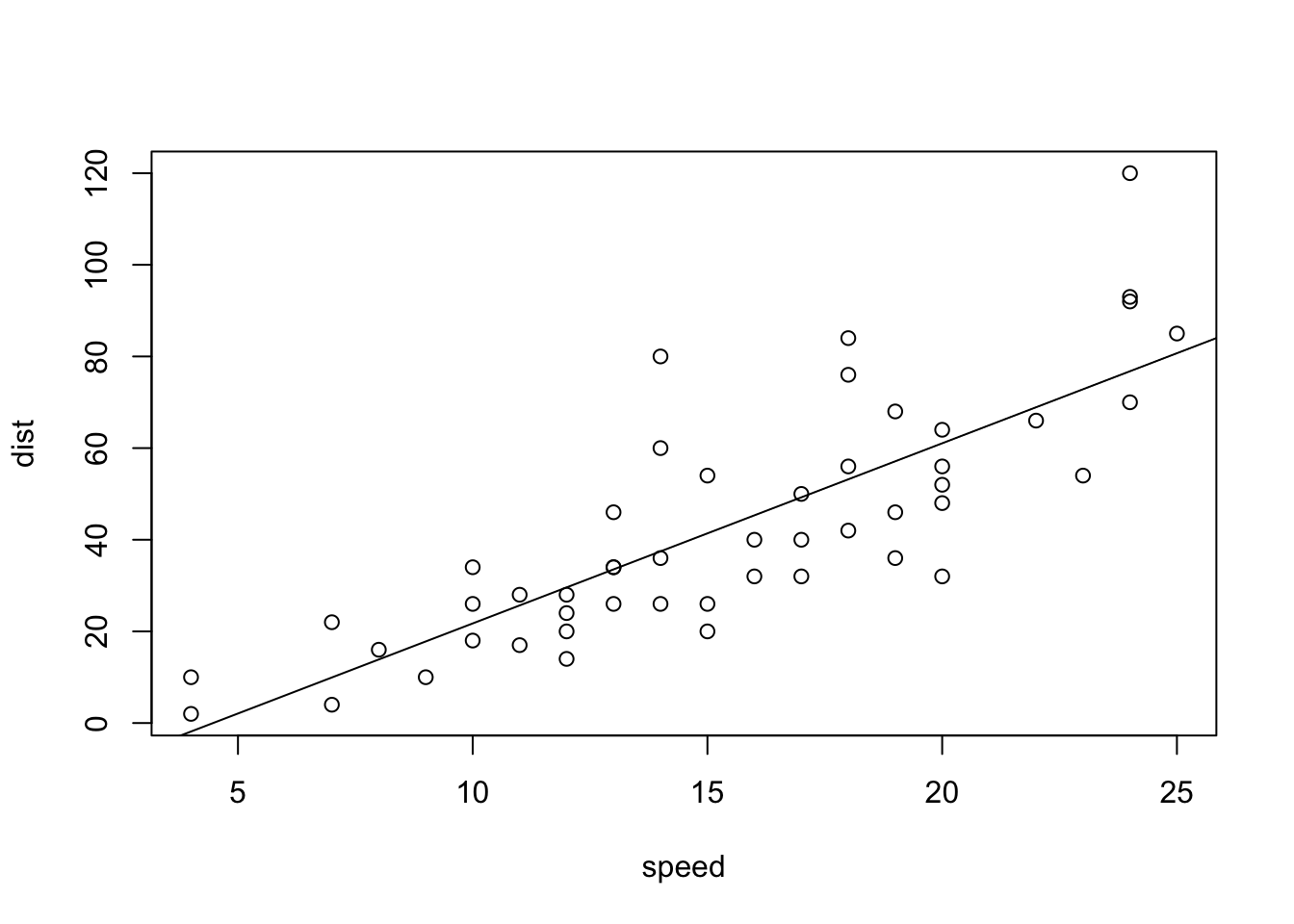``````knitr::opts_chunk\$set(echo = TRUE)
library(tinytex)``````

Source files: [https://github.com/djlofland/DATA605_S2020/tree/master/]

## CARS Analysis

Using the cars dataset in R, build a linear model for stopping distance as a function of speed and replicate the analysis of your textbook chapter 3 (visualization, quality evaluation of the model, and residual analysis.)

``````# ----------- Load cars dataset -----------
data(cars)

cor(cars\$dist, cars\$speed)``````
``##  0.8068949``
``````model <- lm(dist ~ speed, cars)
summary(model)``````
``````##
## Call:
## lm(formula = dist ~ speed, data = cars)
##
## Residuals:
##     Min      1Q  Median      3Q     Max
## -29.069  -9.525  -2.272   9.215  43.201
##
## Coefficients:
##             Estimate Std. Error t value Pr(>|t|)
## (Intercept) -17.5791     6.7584  -2.601   0.0123 *
## speed         3.9324     0.4155   9.464 1.49e-12 ***
## ---
## Signif. codes:  0 '***' 0.001 '**' 0.01 '*' 0.05 '.' 0.1 ' ' 1
##
## Residual standard error: 15.38 on 48 degrees of freedom
## Multiple R-squared:  0.6511, Adjusted R-squared:  0.6438
## F-statistic: 89.57 on 1 and 48 DF,  p-value: 1.49e-12``````
``````plot(dist ~ speed, cars)
abline(model)````````````plot(model\$residuals ~ cars\$speed)
abline(h = 0, lty = 3)  # adds a horizontal dashed line at y = 0``````